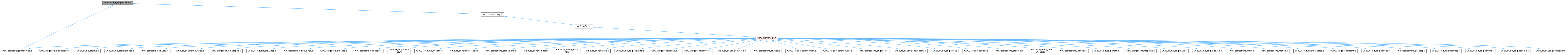ReactOS 0.4.15-dev-6068-g8061a6f
floatobj.h File Reference
This graph shows which files directly or indirectly include this file:Go to the source code of this file.

## Macros

#define FIX2LONG(x)   (((x) + 8) >> 4)

#define LONG2FIX(x)   ((x) << 4)

#define FLOATOBJ_bConvertToLong(pf, pl)   (*pl = (LONG)*pf, TRUE)

#define FLOATOBJ_IsLong(pf)   ((FLOAT)((LONG)*(pf)) == *(pf))

#define FLOATOBJ_Equal0(pf)   (*(pf) == 0.)

#define FLOATOBJ_Equal1(pf)   (*(pf) == 1.)

#define FLOATOBJ_GetFix(pf)   ((LONG)(*(pf) * 16.))

#define FLOATOBJ_0   0.

#define FLOATOBJ_1   1.

#define FLOATOBJ_16   16.

#define FLOATOBJ_1_16   (1./16.)

#define FLOATOBJ_Set0(fo)   *(fo) = 0;

#define FLOATOBJ_Set1(fo)   *(fo) = 1;

## Functions

C_ASSERT (sizeof(FIX)==sizeof(LONG))

## Variables

static const FLOATOBJ gef0 = 0.

static const FLOATOBJ gef1 = 1.

static const FLOATOBJ gef2 = 2.

static const FLOATOBJ gef16 = 16.

## ◆ FIX2LONG

 #define FIX2LONG ( x ) (((x) + 8) >> 4)

Definition at line 4 of file floatobj.h.

## ◆ FLOATOBJ_0

 #define FLOATOBJ_0   0.

Definition at line 108 of file floatobj.h.

## ◆ FLOATOBJ_1

 #define FLOATOBJ_1   1.

Definition at line 109 of file floatobj.h.

## ◆ FLOATOBJ_16

 #define FLOATOBJ_16   16.

Definition at line 110 of file floatobj.h.

## ◆ FLOATOBJ_1_16

 #define FLOATOBJ_1_16   (1./16.)

Definition at line 111 of file floatobj.h.

## ◆ FLOATOBJ_bConvertToLong

 #define FLOATOBJ_bConvertToLong ( pf, pl ) (*pl = (LONG)*pf, TRUE)

Definition at line 102 of file floatobj.h.

## ◆ FLOATOBJ_Equal0

 #define FLOATOBJ_Equal0 ( pf ) (*(pf) == 0.)

Definition at line 104 of file floatobj.h.

## ◆ FLOATOBJ_Equal1

 #define FLOATOBJ_Equal1 ( pf ) (*(pf) == 1.)

Definition at line 105 of file floatobj.h.

## ◆ FLOATOBJ_GetFix

 #define FLOATOBJ_GetFix ( pf ) ((LONG)(*(pf) * 16.))

Definition at line 106 of file floatobj.h.

## ◆ FLOATOBJ_IsLong

 #define FLOATOBJ_IsLong ( pf ) ((FLOAT)((LONG)*(pf)) == *(pf))

Definition at line 103 of file floatobj.h.

## ◆ FLOATOBJ_Set0

 #define FLOATOBJ_Set0 ( fo ) *(fo) = 0;

Definition at line 118 of file floatobj.h.

## ◆ FLOATOBJ_Set1

 #define FLOATOBJ_Set1 ( fo ) *(fo) = 1;

Definition at line 119 of file floatobj.h.

## ◆ LONG2FIX

 #define LONG2FIX ( x ) ((x) << 4)

Definition at line 5 of file floatobj.h.

## ◆ C_ASSERT()

 C_ASSERT ( sizeof(FIX) = `=sizeof(LONG)` )

## ◆ gef0

 const FLOATOBJ gef0 = 0.
static

Definition at line 113 of file floatobj.h.

Referenced by DC_vInitDc().

## ◆ gef1

 const FLOATOBJ gef1 = 1.
static

Definition at line 114 of file floatobj.h.

Referenced by PATH_NormalizePoint(), PATH_ScaleNormalizedPoint(), and ScaleLong().

## ◆ gef16

 const FLOATOBJ gef16 = 16.
static

Definition at line 116 of file floatobj.h.

Referenced by DC_vInitDc().

## ◆ gef2

 const FLOATOBJ gef2 = 2.
static

Definition at line 115 of file floatobj.h.

Referenced by PATH_ScaleNormalizedPoint().